#Algebra 1 Worksheets

## Dynamically Created Algebra 1 Worksheets

Here is a graphic preview for all of the Algebra 1 Worksheet Sections. You can select different variables to customize these Algebra 1 Worksheets for your needs. The Algebra 1 Worksheets are randomly created and will never repeat so you have an endless supply of quality Algebra 1 Worksheets to use in the classroom or at home. We have twelve different topics covering Basic Skills, Domain and Range, Equations, Exponents, Inequalities, Linear Functions, Polynomials, Quadratic Functions, Radical Expressions, Rational Expressions, Systems of Equations, Trigonometry, and Word Problems for your use.

Our Algebra 1 Worksheets are free to download, easy to use, and very flexible.

These Algebra 1 Worksheets are a good resource for students in the 5th Grade through the 8th Grade.

Click here for a Detailed Description of all the Algebra 1 Worksheet Sections.

## Quick Link for All Algebra 1 Worksheet Sections

Click the image to be taken to that Algebra 1 Worksheet Section.

##### Basic SkillsAlgebra 1 Worksheets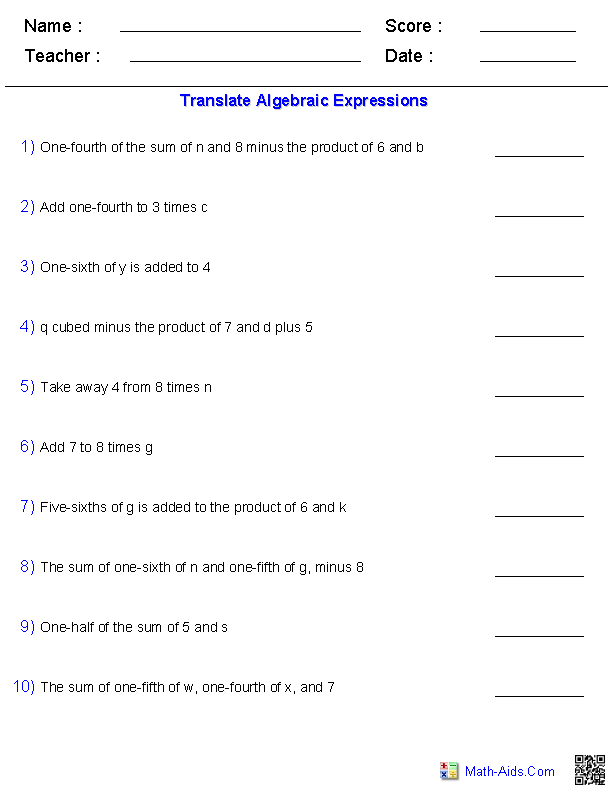##### Domain and RangeAlgebra 1 Worksheets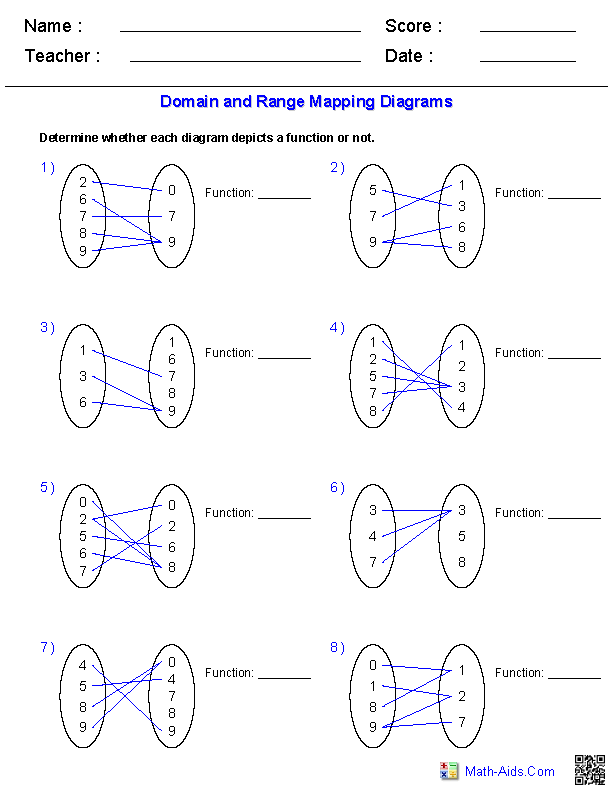##### Equations WorksheetsAlgebra 1 Worksheets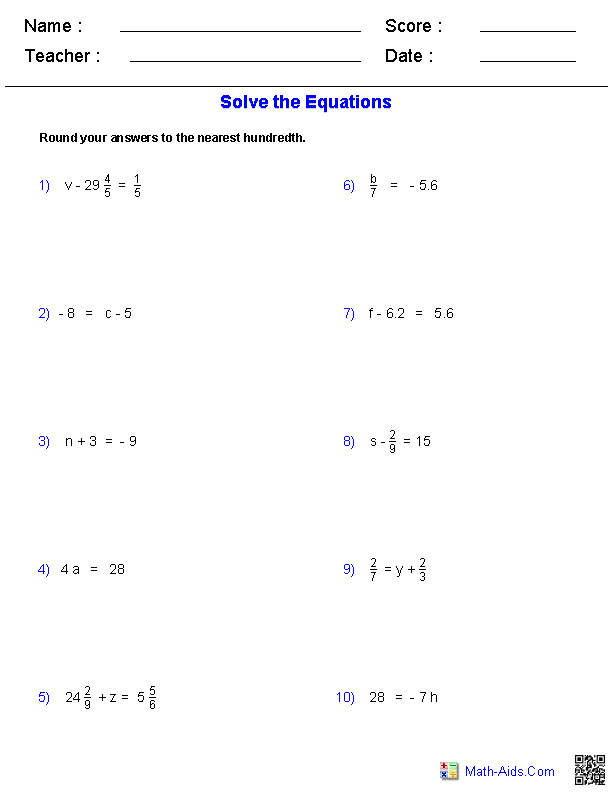##### Exponents WorksheetsAlgebra 1 Worksheets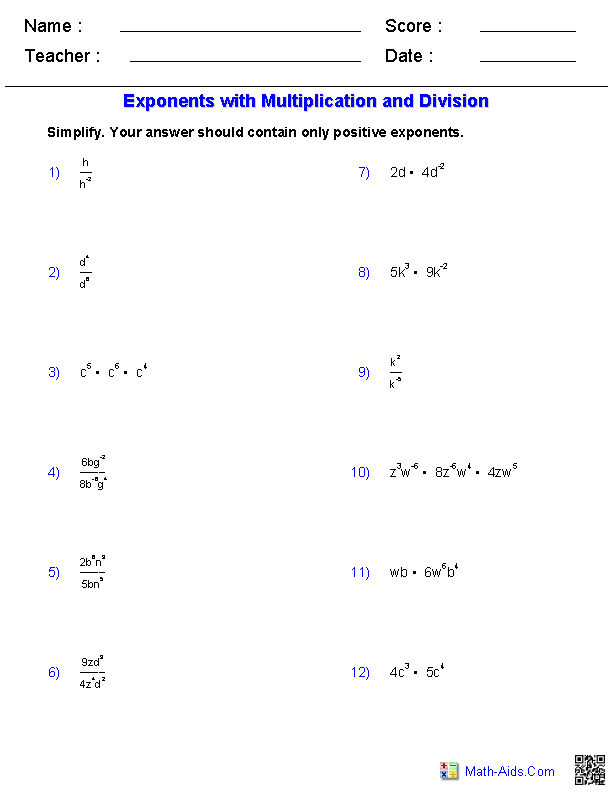##### Inequalities WorksheetsAlgebra 1 Worksheets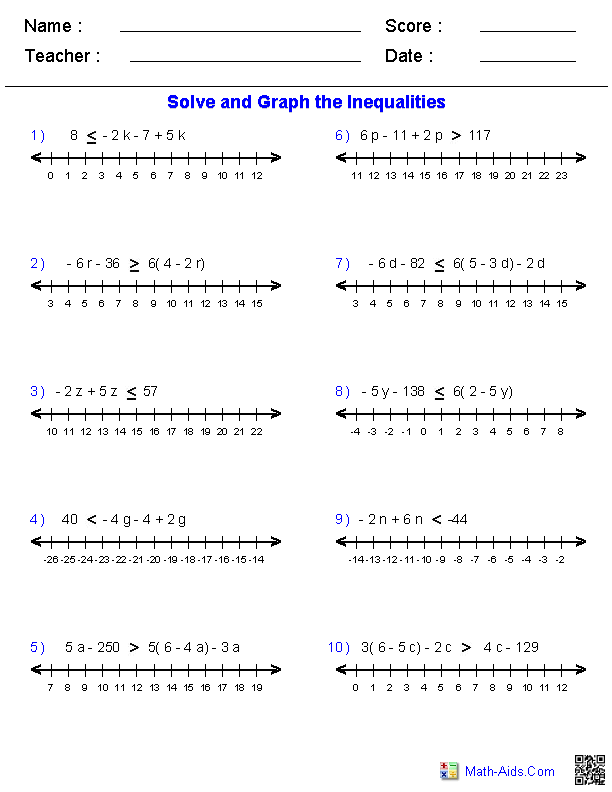##### Linear Equations& InequalitiesAlgebra 1 Worksheets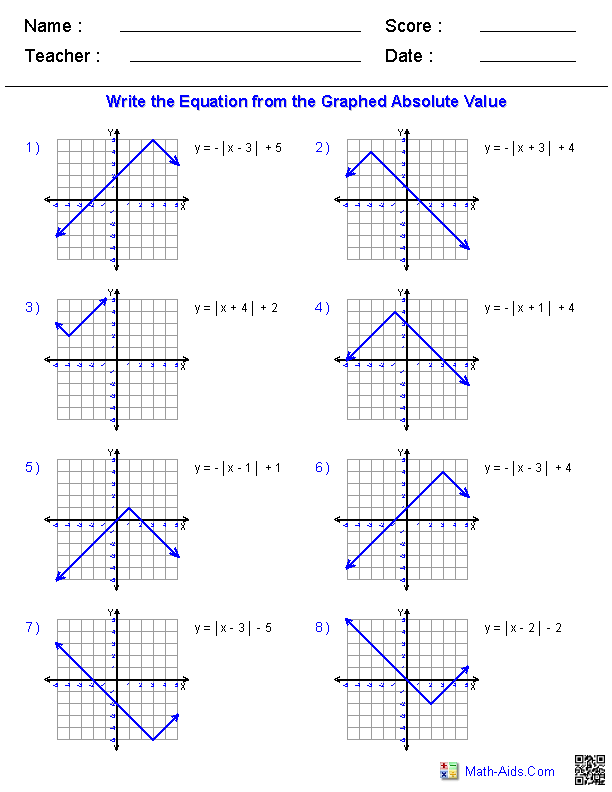##### Monomial and PolynomialsAlgebra 1 Worksheets##### Quadratic Functions WorksheetsAlgebra 1 Worksheets##### Radical Expressions WorksheetsAlgebra 1 Worksheets##### Rational Expressions WorksheetsAlgebra 1 Worksheets##### Systems of EquationsAlgebra 1 Worksheets##### Trigonometry WorksheetsAlgebra 1 Worksheets##### Word ProblemsAlgebra 1 WorksheetsRecommended Videos

## Detailed Description for All Algebra 1 Worksheet Sections

Basic Skills Worksheets
This section contains all of the graphic previews for the Basics for Algebra 1 Worksheets. We currently have worksheets covering writing variable expressions, order of operations, evaluating expressions, number sets, adding and subtracting rational numbers, multiplying and dividing rational numbers, The Distributive Property, combining like terms, and percent of change. These Basics for Algebra 1 Worksheets are a good resource for students in the 7th Grade through the 10th Grade.

Domain and Range Worksheets
This section contains all of the graphic previews for the Domain and Range Worksheets. We currently have worksheets covering identifying functions from mapping diagrams, identifying functions from ordered pairs, identifying functions from graphs, and identifying domains and ranges from graphs. These Domain and Ranges Worksheets are a good resource for students in the 7th Grade through the 10th Grade.

Equations Worksheets
This section contains all of the graphic previews for the Equations Worksheets. We currently have worksheets covering one and two step equations with integers, decimals, and fractions. We also have multi-step equations with integers and decimals. We have equation worksheets that cover absolute values, proportions, and percent problems. We have several word problem in this section such as one and two step equations, "distance, rate, and time" problems, mixture problems, and work word problems. These Equations Worksheets are a good resource for students in the 7th Grade through the 10th Grade.

Exponents Worksheets
This section contains all of the graphic previews for the Exponents Worksheets. We currently have worksheets covering exponents properties handout, evaluating exponents, graphing exponents functions, exponents with multiplication, exponents with division, powers of products, powers of quotients, operations with exponents, writing scientific notation, and operations with scientific notation. These Exponents Worksheets are a good resource for students in the 7th Grade through the 10th Grade.

Inequalities Worksheets
This section contains all of the graphic previews for the Inequalities Worksheets. We currently have worksheets covering inequality properties handout, graphing single variable inequalities, one step inequalities by adding and subtracting, one step inequalities by multiplying and dividing, two step inequalities, multiple step inequalities, compound inequalities, and absolute value inequalities. These Inequalities Worksheets are a good resource for students in the 7th Grade through the 10th Grade.

Linear Equations Worksheets
This section contains all of the graphic previews for the Linear Equations Worksheets. We currently have worksheets covering finding slope from a graphed line, find slope from a pair of points, finding slope and y-intercept from a linear equation, graphing lines in slope-intercept form, graphing lines in standard form, working with linear equations, writing linear equations, graphing linear inequalities, and graphing absolute values. These Linear Equations Worksheets are a good resource for students in the 7th Grade through the 10th Grade.

Monomials and Polynomials Worksheets
This section contains all of the graphic previews for the Monomials and Polynomials Worksheets. We currently have worksheets covering identifying types of monomials and polynomials, identifying the degree of monomials and polynomials, naming of monomials and polynomials, adding and subtracting monomials and polynomials, multiplying monomials and polynomials, multiplying special case polynomials, dividing polynomials, factoring quadratic polynomials, factoring special case polynomials, and factoring by grouping polynomials. These Monomials and Polynomials Worksheets are a good resource for students in the 7th Grade through the 10th Grade.

This section contains all of the graphic previews for the Quadratic Functions Worksheets. We currently have worksheets covering graphing quadratic functions, graphing quadratic inequalities, and completing the square. We also have worksheets for solving quadratic equations by taking the square root, by factoring, with the quadratic formula, and by completing the square. These Quadratic Functions Worksheets are a good resource for students in the 7th Grade through the 10th Grade.

This section contains all of the graphic previews for the Radical Expressions Worksheets. We currently have worksheets covering simplifying radicals expressions, adding and subtracting radicals expressions, multiplying radicals expressions, dividing radicals expressions, solving radical equations, using the distance formula, and using the midpoint formula. These Radical Expressions Worksheets are a good resource for students in the 7th Grade through the 10th Grade.

Rational Expressions Worksheets
This section contains all of the graphic previews for the Rational Expressions Worksheets. We currently have worksheets covering simplifying rational expressions, adding and subtracting rational expressions, multiplying rational expressions, dividing rational expressions, and solving rational equations. These Rational Expressions Worksheets are a good resource for students in the 7th Grade through the 10th Grade.

System of Equations Worksheets
This section contains all of the graphic previews for the System of Equations Worksheets. We currently have worksheets covering handout for systems of equations, solving algebraically two variable systems of equations, solving graphically two variable systems of equations, and solving graphically two variable systems of inequalities. These System of Equations Worksheets are a good resource for students in the 7th Grade through the 10th Grade.

Trigonometry Worksheets
This section contains all of the graphic previews for the Trigonometry Worksheets. We currently have worksheets covering trigonometric rations, inverse trigonometric ratios, solving right triangles, and multi-step problems. These Trigonometry Worksheets are a good resource for students in the 7th Grade through the 10th Grade.

Word Problems Worksheets
This section contains all of the graphic previews for the Word Problems Worksheets. We currently have worksheets covering one and two step equations, "distance, rate, and time" problems, mixture problems, and work problems. These Word Problems Worksheets are a good resource for students in the 7th Grade through the 10th Grade.